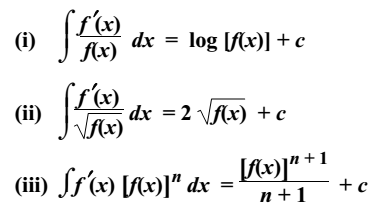# Integration by Substitution

Sometimes, the given functions may not be in an integration form and the  variable of integration (x in dx) can be suitably changed into a new variable by substitution so that the new function can be integrated. Integration by Substitution is also called u-substitution.

Let F(u) = ∫ f(u) du, then

dF(u)/du = f(u)

If u = φ(x), then

du/dx = φ′(x)

dF(u)/dx = dF(u)/du . du/dx

= f(u) φ′(x)

dF(u)/dx = f[φ(x)] φ′(x)

⇒ F(u) = ∫ f[φ(x)] φ′(x) dx

∫ f(u)du = ∫ f[φ(x)] φ′(x) dx

### Standard Results of Integrals